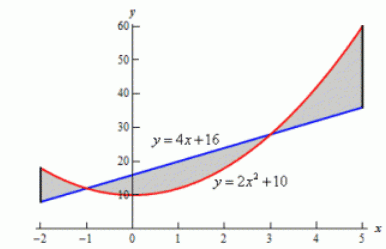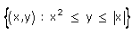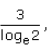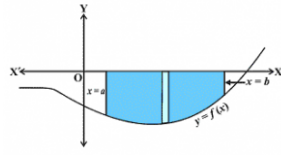# Test: Area Between A Curve And A Line

## 10 Questions MCQ Test Mathematics (Maths) Class 12 | Test: Area Between A Curve And A Line

Description
This mock test of Test: Area Between A Curve And A Line for JEE helps you for every JEE entrance exam. This contains 10 Multiple Choice Questions for JEE Test: Area Between A Curve And A Line (mcq) to study with solutions a complete question bank. The solved questions answers in this Test: Area Between A Curve And A Line quiz give you a good mix of easy questions and tough questions. JEE students definitely take this Test: Area Between A Curve And A Line exercise for a better result in the exam. You can find other Test: Area Between A Curve And A Line extra questions, long questions & short questions for JEE on EduRev as well by searching above.
QUESTION: 1

### The area enclosed between the lines x = 2 and x = 7 is​

Solution:

Area enclosed between the lines x = 2 and x = 7 is Infinite.

QUESTION: 2

Solution:
QUESTION: 3

### Area under the circle x2 + y2 = 16 is​

Solution:
QUESTION: 4

Area of the shaded region in the given figure is :Solution:
QUESTION: 5

Area of the region bounded by the curve y2 = 2y – x and y-axis is:​

Solution:

y= 2y−x
⇒ x = 2y − y2
Curve meets y−axis where x= 0
2y − y= 0
⇒ y (2−y) = 0
y = 0 or y = 2
Area =∣∫(0 to 2) x.dy∣
=|∫(0 to 2) (2y − y2).dy∣
=∣[y2 − y3/3] (0 to 2) |[4−8/3]−0|
=4/3 unit2

QUESTION: 6

The area of the region bounded between the line x=9 and the parabola y2=16x is​

Solution:

Explanation : Equation of the parabola is

y2 = 16x .... (i)

Required area =2∫(0 to 9) ydx

= 2∫(0 to 9) 4(x)1/2 dx

= 8∫(0 to 9) (x)1/2 dx

​= 8[(x3/2)/(3/2)](0 to 9)

​= 16/3[x3/2](0 to 9)

= 144 sq unit.

QUESTION: 7

Area of the regionis :

Solution:
QUESTION: 8

If the area above x-axis, bounded by the curves y = 2kx, x = 0 and x = 2 isthen k = ?​

Solution:
QUESTION: 9

Write the shaded region as an integralSolution:
QUESTION: 10

The area bounded by the curve:

y = cos2 x between x = 0, x = π and x axis

Solution:

y = cos2 x [0,π]
∫(0 to π/2)cos2 xdx + ∫(π/2 to π)cos2 xdx
= 1/2∫(1 + cos 2x)
= [½(x + sin(2x)/2](0 to π/2] + [1/2(x + sin(2x)/2](π/2 to π)
= ½|π + 0 - 0 - 0| + 1/2|(π + 0) - π/2|
= π/4 + π/4 = π/2